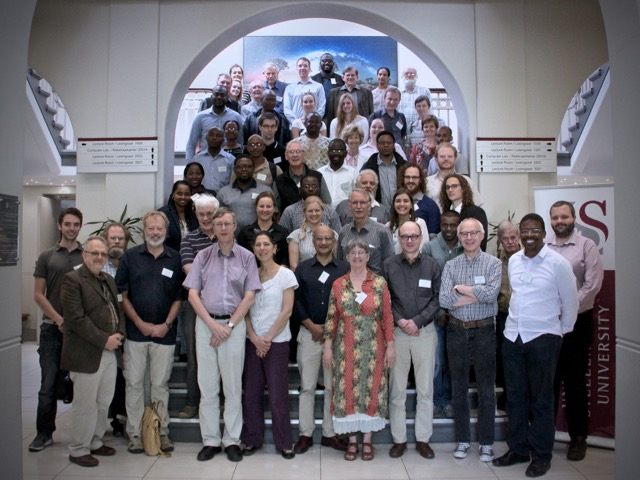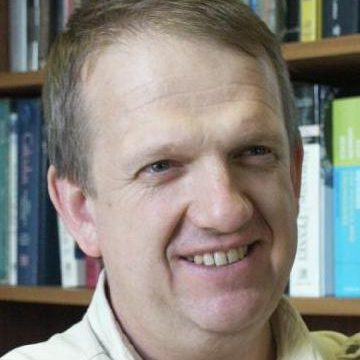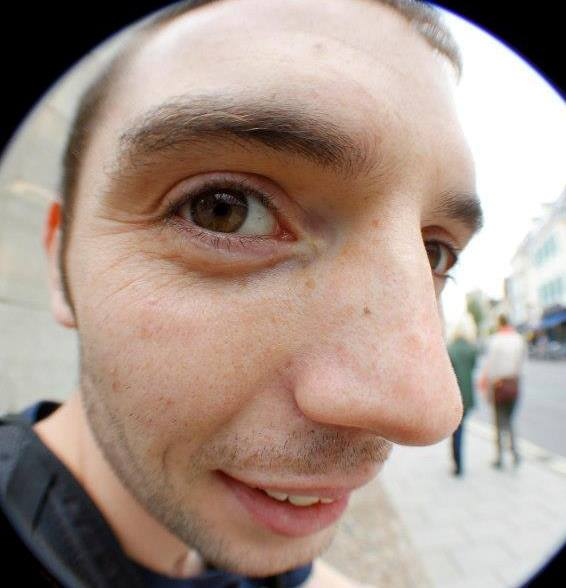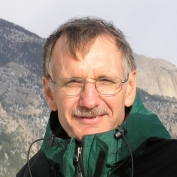SANUM2016
Stellenbosch University
22nd to 24th March

#### Thank you, conference photo, and slides

The organisers would like to say a huge thank you to everyone who attended SANUM 2016, we think it was a huge success!There were a number of requests to make the slides from the talks available online, and many of these can be found here. Unfortunately the slides from the upstairs were removed before they could be copied over. We ask anyone who presented in that room (or anyone else whose slides do not appear in this list) to email them to at sanum2016@gmail.com so that they might be included.

#### SANUM 2016

The 40th South African Symposium of Numerical and Applied Mathematics (SANUM) will take place at the University of Stellenbosch from 22nd to 24th March 2016.

SANUM has a long standing tradition as an event in the scientific life in South Africa. Following in this tradition, the scope of SANUM 2016 includes, but is not limited to, the following topics:

• Ordinary differential equations, modelling, associated numerical analysis
• Partial differential equations, modelling, associated numerical analysis
• Numerical Analysis
• Biomathematics
• Image analysis
• Optimization
• Approximation theory

#### Plenary speakers

• Mapundi Banda (University of Pretoria)
• Optimal control and inverse problems for evolution models
Optimal control and inverse problems governed by partial differential equations will be considered. The first part of the presentation will derive continuous and discrete schemes for optimal control problems subject to nonlinear, hyperbolic conservation laws. The initial condition will be treated as the control and a tracking-type problem also with non-smooth desired states will be approximated. The second part will consider a simultaneous identification of a coefficient and an initial condition of a partial differential equation from boundary measurement problem. An identification algorithm will be discussed. Some numerical simulations to show the effectiveness of the proposed algorithm will also be demonstrated.
• Folkmar Bornemann (TU Munich)
• Numerical Problems Inspired by Discrete Complex Analysis
Discrete Complex Analysis, that is, the quest for discretizing the whole theory and not just single manifestations of it has led to a wealth of interesting mathematical concepts and a rich nonlinear theory related to integrable systems. A major question is the construction of discrete analogues of the set of "standard" holomorphic maps and a good model problem is the power map z^a. The stable numerical evaluation of that map touches on many mathematical topics ranging from boundary value problems of discrete Painlevé equations to infinite-dimensional linear algebra and discrete optimization.
• Nick Higham (University of Manchester)
• Challenges in Multivalued Matrix Functions

Multivalued matrix functions arise in solving various kinds of matrix equations. The matrix logarithm is the prototypical example. Another example is the Lambert W function of a matrix, which is much less well known but has been attracting recent interest. A theme of the talk is the importance of choosing appropriate principal values and making sure that the correct choices of signs and branches are used, both in theory and in computation. We will give examples where incorrect results have previously been obtained.

We focus on matrix inverse trigonometric and inverse hyperbolic functions, beginning by investigating existence and characterization. Turning to the principal values, various functional identities are derived, some of which are new even in the scalar case, including a "round trip'' formula that relates acos(cos A) to A and similar formulas for the other inverse functions. Key tools used in the derivations are the matrix unwinding function and the matrix sign function.

A new inverse scaling and squaring type algorithm employing a Schur decomposition and variable-degree Padé approximation is derived for computing acos, and it is shown how it can also be used to compute asin, acosh, and asinh. In numerical experiments the algorithm is found to behave in a forward stable fashion and to be superior to computing these functions via logarithmic formulas.

• Elizabeth Mansfield (University of Kent)
• Lattice based Multispace and Applications

In this talk I will describe the construction of a manifold called "multispace" which uses the Lagrange interpolation data of functions as coordinates and which has the jet space for functions embedded as a sub manifold.

The purpose for the construction is the simultaneous calculation of smooth and discrete invariants of Lie group actions on the base space, via a moving frame, together with their differential (smooth) and recurrence (discrete) relations, with all appropriate continuum limits guaranteed by the construction.

Two main applications have been described to date, to discrete integrable systems and to discrete variational systems. I will discuss the application to variational shallow water systems, showing how a simultaneous smooth and discrete

Noether's theorem leads to the conservation of energy and momenta in the discrete scheme, with guaranteed continuum limits to the smooth energy and momenta.

This is joint work with Gloria Mari Beffa (U Wisconsin-Madison).

• Beatrice Pelloni (University of Reading)
• Beyond integrability: the far-reaching consequences of thinking about boundary conditions

In this talk, I will outline results obtained in the last fifteen years, all stemming from the original aim to include boundary conditions into the celebrated Inverse Scattering Transform, which is in essence a nonlinear Fourier transform.

The talk will revisit the Fourier transform on R, embedding it in a general way of thinking about integral transform that relies on a formulation in the complex domain (called a Riemann-Hilbert formulation) and start from this idea to describe a generalised approach, now known as the unified transform, or Fokas transform. This circle of ideas has produced unexpected and very general results for the solution of linear boundary value problems, including interface and moving boundary problems, new ideas for numerical schemes, a way to study of nonselfadjoint differential operators, as well as going some way to achieving the original aim of solving nonlinear integrable problems in domains with boundaries.

I will describe the key ideas and some of the most unexpected results.

• Daya Reddy (University of Cape Town)
• Discontinuous Galerkin Methods: An Overview and Some Applications

Discontinuous Galerkin (DG) methods, as the name suggests, are a class of Galerkin finite element methods in which the condition of continuity of functions across element boundaries is relaxed. DG methods have become a powerful tool in the approximate solution of hyperbolic problems, the context in which they were first developed. DG methods have also been applied with much success to elliptic PDEs and systems, and it is this class of applications that will be the focus of this presentation. A simple model problem will serve as the vehicle for motivating the DG approach and comparing it to the conventional finite element method. A further application will be in the context of problems arising in solid and fluid mechanics, and in which dependence on a parameter may lead to singular behaviour of the approximate solution. It will be shown how uniformly convergent DG approaches can be constructed in such situations.

• Nick Trefethen (University of Oxford)

Quadrature is the term for the numerical evaluation of integrals. It's a beautiful subject because it's so accessible, yet full of conceptual surprises and challenges. This talk will review ten of these, with plenty of history and numerical demonstrations. Some are old if not well known, some are new, and two are subjects of my current research.

• Walter Van Assche (KU Leuven)

The talk will be about simultaneous Gaussian quadrature (introduced by Borges in 1994) for two integrals of the same function f but on two disjoint intervals. The quadrature nodes are zeros of a type II multiple orthogonal polynomial for an Angelesco system. We recall some known results for the quadrature nodes and the quadrature weights and prove some new results about the convergence of the quadrature formulas. Furthermore we give some estimates of the quadrature weights. Our results are based on a vector equilibrium problem in potential theory and weighted polynomial approximation. This is joint work with Doron Lubinsky (Georgia Institute of Technology, Atlanta GA, USA).

#### Special sessions

We are pleased to announce the following special sessions:

• Orthogonal Polynomials, Special Functions, and Applications

We invite delegates to submit abstracts for a special session on all aspects of orthogonal polynomials and special functions including asymptotics, approximation theory, continued fractions, integrable systems, interpolation and quadrature; Painlevé equations, probability theory and statistics, q-Calculus, Riemann-Hilbert problems, spline functions of one and several variables; stochastic processes, wavelet theory and its applications in signal and image processing as well as practical applications and computational analysis related to any of the above.

• Machine Learning and Computer Vision

Confirmed speakers in this session:

• Ulrich Paquet, Apple research
• On the Foundations and Future of Approximate Inference
• New ideas on Neural Networks and Probabilistic Graphical Models
• Johan du Preez, Electronic Engineering, University of Stellenbosch
• Super pixels and Probabilistic Graphical Models
• Probabilistic Graphical Models for solving the Four Colour problem
• Ben Herbst, Applied Mathematics, University of Stellenbosch
• Probabilistic Graphical Models and Semantic Image Segmentation
• Emli-Mari Nel, Formerly of David Mackay's Inference Group, University of Cambridge.
• Title to be announced
• Bruce Bassett, AIMS and University of Cape Town.
• Title to be announced

• Modelling ecological and evolutionary dynamics

Ecological and evolutionary dynamics can be complex. Mathematical models and techniques that can capture and predict the dynamic behaviour of ecological and evolutionary systems as well as how these systems respond to change are advancing at a rapid pace. These mathematical and numerical tools are crucial for efficiently addressing the global environmental challenges we facing today. This session brings together a diverse talks on how to model dynamics of ecological and evolutionary systems, addressing issues from trait evolution to biological invasions, from community assemblage rules to network emergence.

#### Important dates

• 22nd Feb - Early bird registration ends
• 1st Mar - Deadline for titles and abstracts

#### Organising committee#### André Weideman

weideman@sun.ac.za#### Nick Hale

nickhale@sun.ac.zaherbst@sun.ac.za